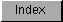```
The FFP basic math library contains entries for the basic mathematics
functions such as add, subtract and divide.  It resides in ROM and is
opened by calling OpenLibrary() with "mathffp.library" as the argument.

#include <exec/types.h>
#include <libraries/mathffp.h>

#include <clib/mathffp_protos.h>

struct Library *MathBase;

VOID main()
{
if (MathBase = OpenLibrary("mathffp.library", 0))
{
. . .

CloseLibrary(MathBase);
}
else
printf("Can't open mathffp.library\n");
}

The global variable MathBase is used internally for all future library
references.

FFP Basic Functions

```